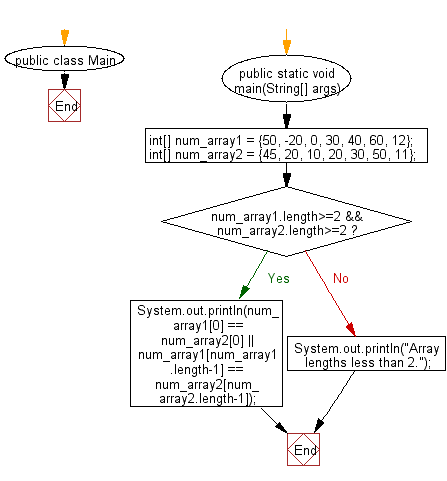﻿ Java: Test if the first or last values of 2 arrays are same# Java Exercises: Test if the first or the last elements of two arrays of integers are same

## Java Basic: Exercise-76 with Solution

Write a Java program to test if the first or the last elements of two arrays of integers are same. The length of the two arrays must be greater than or equal to 2.

Sample Solution:

Java Code:

``````public class Main {
public static void main(String[] args)
{
//false
int[] num_array1 = {50, -20, 0, 30, 40, 60, 12};
int[] num_array2 = {45, 20, 10, 20, 30, 50, 11};

//true
//int[] num_array1 = {50, -20, 0, 30, 40, 60, 12};
//int[] num_array2 = {45, 20, 10, 20, 30, 50, 12};

//Array lengths less than 2.
//int[] num_array1 = {50};
//int[] num_array2 = {45};

if(num_array1.length>=2 && num_array2.length>=2)
{
System.out.println(num_array1 == num_array2 || num_array1[num_array1.length-1] == num_array2[num_array2.length-1]);
}
else
{
System.out.println("Array lengths less than 2.");
}
}
}
```
```

Sample Output:

```false
```

Pictorial Presentation:Flowchart:Java Code Editor:

What is the difficulty level of this exercise?

Test your Programming skills with w3resource's quiz.

﻿

## Java: Tips of the Day

countOccurrences

Counts the occurrences of a value in an array.

Use Arrays.stream().filter().count() to count total number of values that equals the specified value.

```public static long countOccurrences(int[] numbers, int value) {
return Arrays.stream(numbers)
.filter(number -> number == value)
.count();
}
```

Ref: https://bit.ly/3kCAgLb